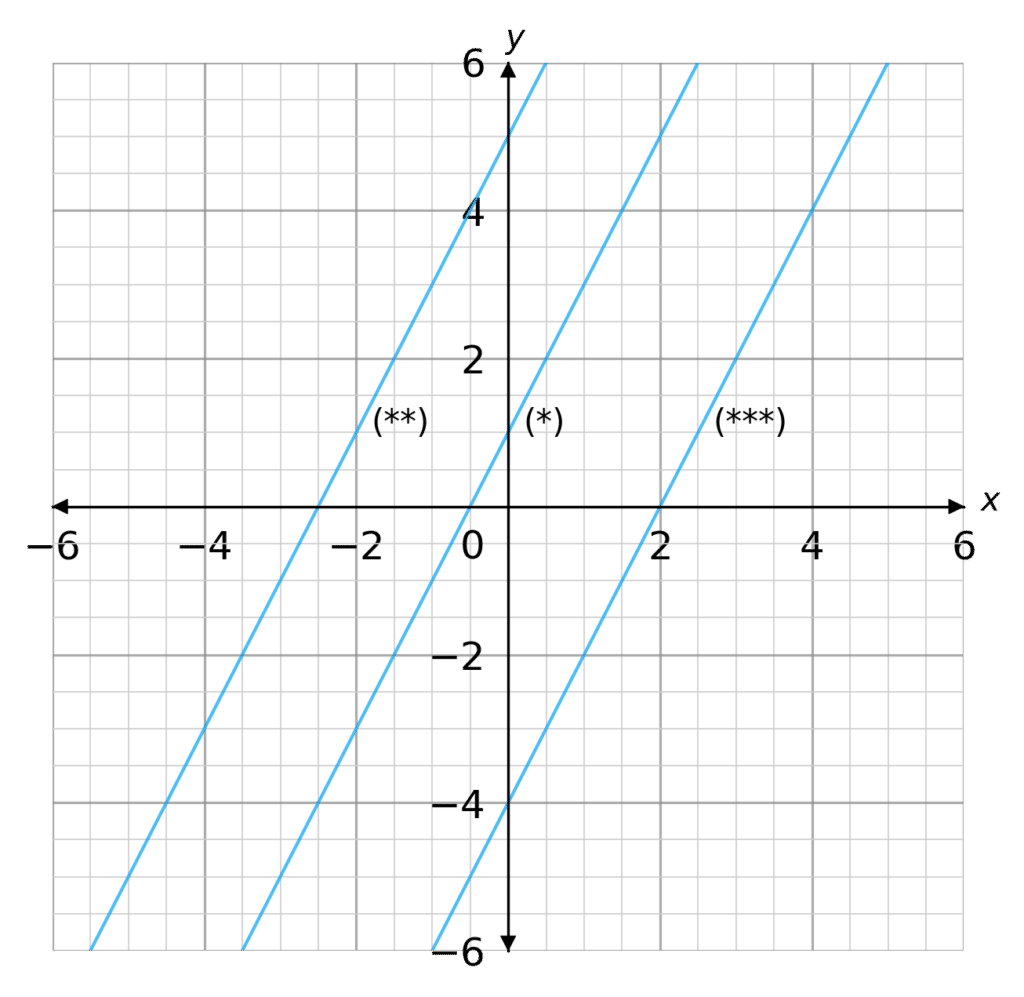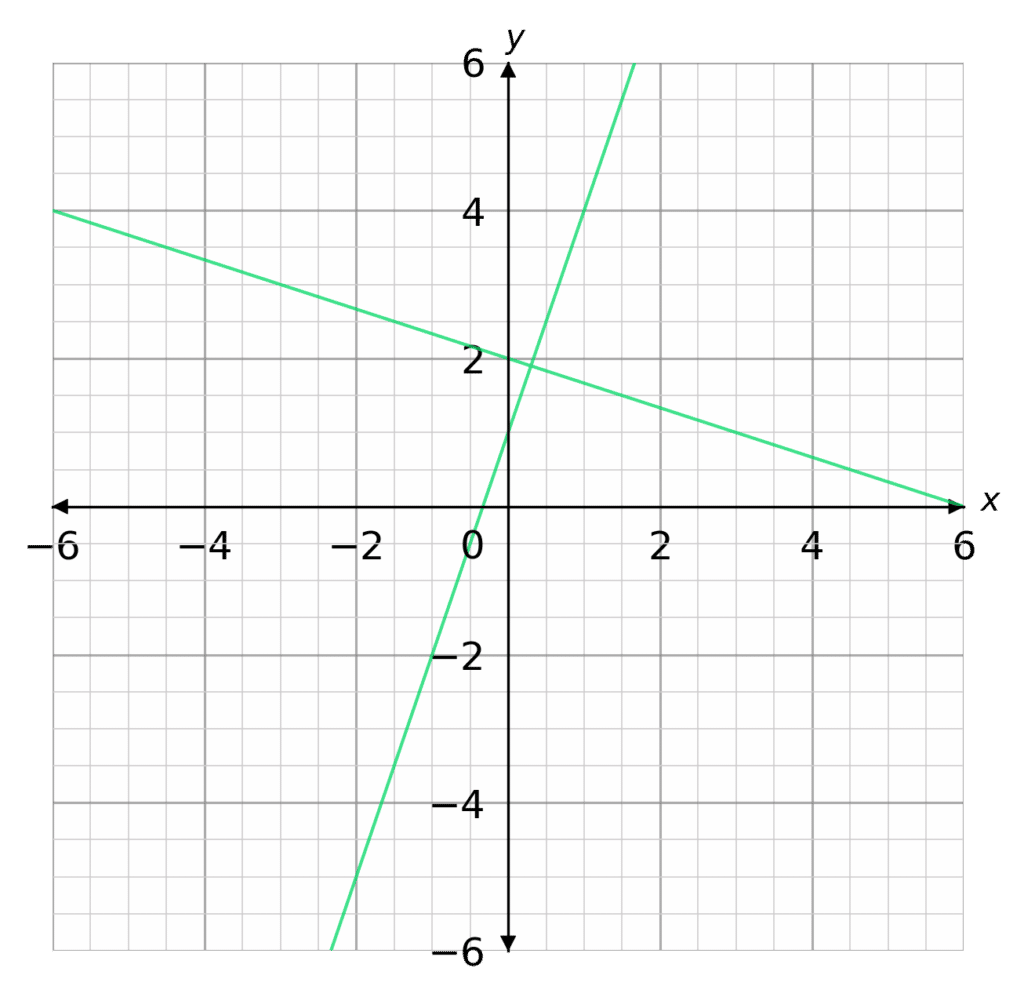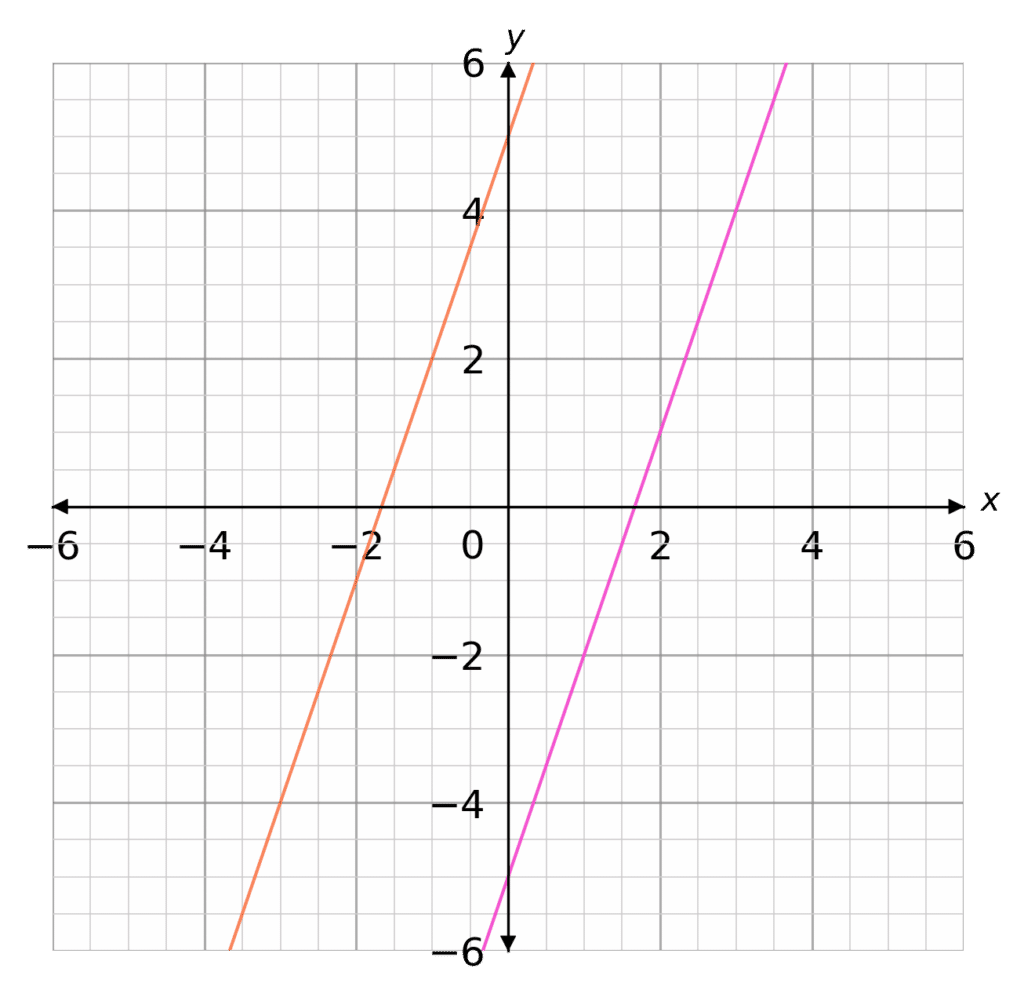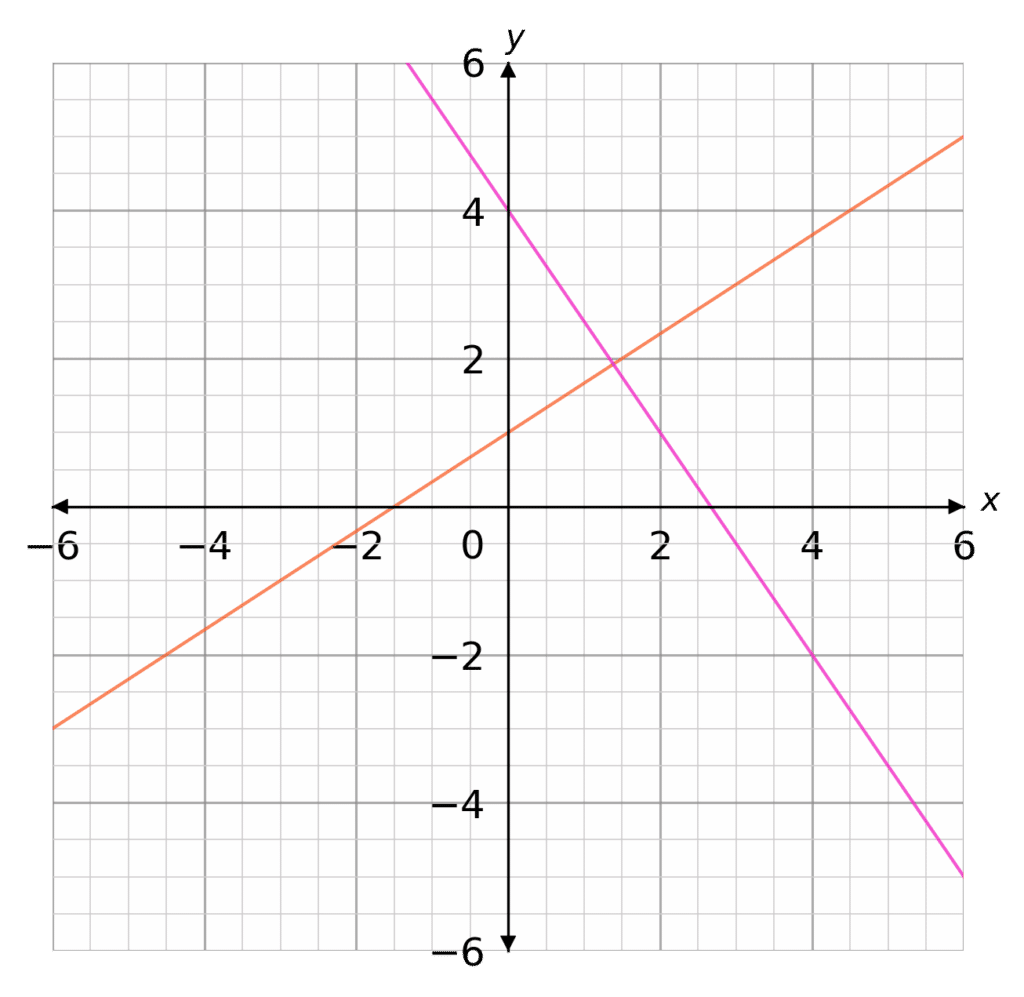# Parallel and Perpendicular Lines

GCSELevel 6-7Edexcel iGCSE

## Parallel and Perpendicular Lines

Parallel Lines – are always the same distance apart and never meet (they have the same gradient).

Perpendicular Lines – meet at a right angle (the gradients of perpendicular lines multiply to $-1$).

Before continuing this topic, recap the following:

## Parallel Lines – Same GradientParallel lines have the same gradient.

Equation of a straight line: $y=\textcolor{#10a6f3}{m}x+c$

$\textcolor{#10a6f3}{m} \rightarrow$ gradient

$c \rightarrow y-$ intercept

For lines to be parallel, the value of $\textcolor{#10a6f3}{m}$ must be the same.

Let’s take a look at the following lines:

$y=\textcolor{#10a6f3}{2}x+1$ (*)

$y\textcolor{#10a6f3}{-2}x=5$ (**)

$y=\textcolor{#10a6f3}{2}x -4$ (***)

The second equation (**) must be rearranged into the form

$y=\textcolor{#10a6f3}{m}x+c$, before comparing the gradients:

$y=\textcolor{#10a6f3}{2}x+5$

These lines all have a gradient of $\textcolor{#10a6f3}{2} \, (m=2)$. These straight line equations are shown on the graph to the right.

Level 6-7GCSEEdexcel iGCSE

## Perpendicular Lines – Right AnglesPerpendicular lines meet at a right angle. When we multiply the gradients of a pair of perpendicular lines they multiply to $\textcolor{#00d865}{-1}$.

Another way of looking at this concept is to think of the perpendicular gradient as the ‘opposite sign and reciprocal’.

For instance, let’s say we have a straight line with a gradient: $\textcolor{#00d865}{m=2}$

Then the perpendicular gradient would be: $\textcolor{#00d865}{m=-\dfrac{1}{2}}$

$\textcolor{#00d865}{2}\times\textcolor{#00d865}{-\dfrac{1}{2}}=\textcolor{#00d865}{-1}$

Let’s take a look at the following lines:

$y=\textcolor{#00d865}{3}x+1$

$y=\textcolor{#00d865}{-\dfrac{1}{3}}x+2$

These lines are perpendicular as: $\textcolor{#00d865}{3}\times\textcolor{#00d865}{-\dfrac{1}{3}}=\textcolor{#00d865}{-1}$. These straight line equations are shown on the graph to the right.

Level 6-7GCSEEdexcel iGCSE

## Example 1: Parallel LinesFind the equation of a straight line that is parallel to $\textcolor{#f95d27}{y=3x+5}$ and passes through $(1,-2)$

Equation of the parallel line: $y=3x+c$

[3 marks]

$c$ is the $y \,$intercept and can take any value. However, because we know the line passes through the coordinate $(1,-2)$, we can find the exact value of $c$

To do this, we substitute $x=1$ and $y=-2$

$-2=3(1)+c \rightarrow c=-5 \rightarrow \textcolor{#f21cc2}{y=3x-5}$
Level 6-7GCSEEdexcel iGCSE

## Example 2: Perpendicular LinesFind the equation of a straight line that is perpendicular to

$\textcolor{#f95d27}{y=\dfrac{2}{3}x+1}$ and passes through $(2,1)$

[3 marks]

Equation of the perpendicular line: $y=-\dfrac{3}{2}x+c$

$c$ is the $y \,$intercept and can take any value. However, because we know the line passes through the coordinate $(2,1)$, we can find the exact value of $c$

To do this, we substitute $x=2$ and $y=1$

$1=-\dfrac{3}{2}(2)+c \rightarrow c=4 \rightarrow \textcolor{#f21cc2}{y=-\dfrac{3}{2}x+4}$
Level 6-7GCSEEdexcel iGCSE

## Parallel and Perpendicular Lines Example Questions

After some rearrangement, to get the equations in the form of $y=mx+c$, we get the following:

a) $y=2x+5$

b) $y=-3x+6$

c) $y=2x-3$

d) $y=\dfrac{1}{3}x+9$

a) and c) are parallel, as the gradients are the same.

b) and d) are perpendicular, as when you multiply the gradients together you get $-1$.

We first need to rearrange the equation into the form of  $y=mx+c$:

$y=8x+11$

Equation of parallel line:

$y=8x+c$

Substituting $x=2$ and $y=5$

$5=8(2)+c \rightarrow c=-11 \rightarrow y=8x-11$

We first need to rearrange the equation into the form of $y=mx+c$:

$y=-\dfrac{1}{5}x+10$

Equation of perpendicular line:

$y=5x+c$

Substituting $x=2$ and $y=12$

$12=5(2)+c \rightarrow c=2 \rightarrow y=5x+2$

## You May Also Like...### MME Learning Portal

Online exams, practice questions and revision videos for every GCSE level 9-1 topic! No fees, no trial period, just totally free access to the UK’s best GCSE maths revision platform.

£0.00